Courses

# RD Sharma Solutions - Ex-6.2, Exponents, Class 7, Math Class 7 Notes | EduRev

## Class 7: RD Sharma Solutions - Ex-6.2, Exponents, Class 7, Math Class 7 Notes | EduRev

The document RD Sharma Solutions - Ex-6.2, Exponents, Class 7, Math Class 7 Notes | EduRev is a part of the Class 7 Course RD Sharma Solutions for Class 7 Mathematics.
All you need of Class 7 at this link: Class 7

Q1. Using laws of exponents, simplify and write the answer in exponential form

(i) 23×24×25

(ii) 512÷53

(iii) (72)3

(iv) (32)5÷34

(v) 37×27

(vi) (521÷513)×57

Sol:

(i) 23×24×25

We know that, am+an+a= am+n+p

So, 23×24×25 = 23+4+5

= 212

(ii) 512÷53

We know that, am÷a= am−n

So, 512÷53 = 512−3

= 59

(iii) (72)3

We know that, (am)n=amn

So, (72)3 = 7(2)(3)

= 76

(iv) (32)5÷34

We know that, am÷a= am−n and (am)= amn

So, (32)5÷34 = 310÷34

= 310−4

= 36

(v) 37×27

We know that, (am×bm)=(a×b)m

So, 37×27 = (3×2)7

= 67

(vi) (521÷513)×57

We know that, am÷an=am−n and(am×an)=(a)m+n

So, (521÷513)×57 = (521−13)×57

= (58)×57

= 58+7

= 515

Q2. Simplify and express each of the following in exponential form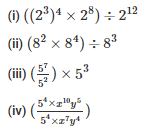Sol: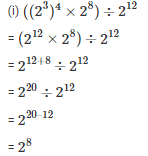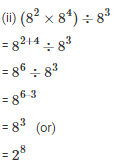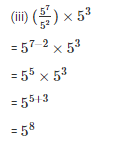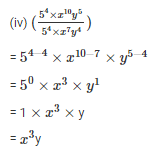Q3. Simplify and express each of the following in exponential form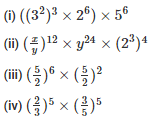Sol: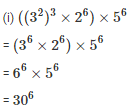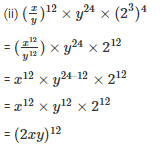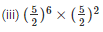We know that,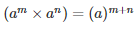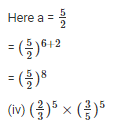We know that,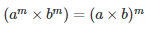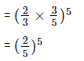Q4. Write 9 ×9 ×9 ×9 ×9 in exponential form with base 3

Sol:

9 ×9 ×9 ×9 ×9 = (9)5 = (32)5

= 310

Q5. Simplify and write each of the following in exponential form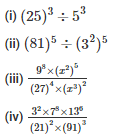Sol: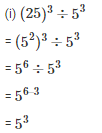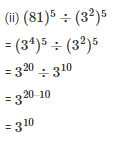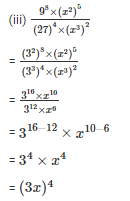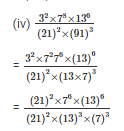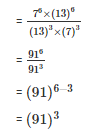Q6. Simplify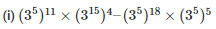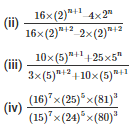Sol: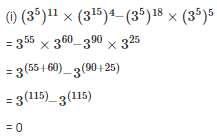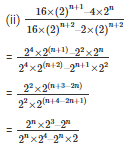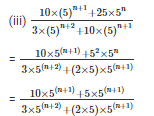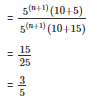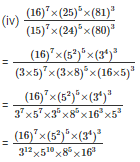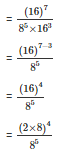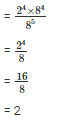Q7. Find the values of n in each of the following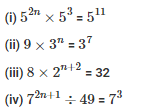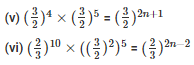Sol: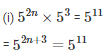Equating the powers

= 2n + 3 = 11

= 2n = 11- 3

=  2n = 8

= n = 4

(ii) 9×3n = 37

= 32×3n = 37

= 32+n = 37

Equating the powers

= 2 + n = 7

= n = 7 – 2

= n = 5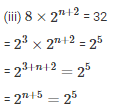Equating the powers

= n + 5 = 5

= n = 0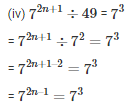Equating the powers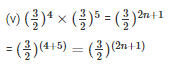Equating the powers

= 4 + 5 = 2n + 1

= 2n + 1 = 9

= 2n = 8

= n = 4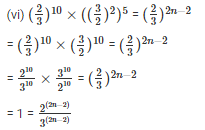= 3(2n – 2) =2(2n – 2)

= 6n – 6 = 4n – 4

= 6n – 4n = 6 – 4

= 2n = 2

= n = 1

Q8.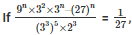find the value of n

Sol: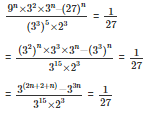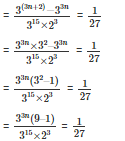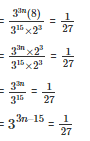On equating the coefficient

3n – 15 = -3

3n = -3 + 15

3n = 12

n = 4

The document RD Sharma Solutions - Ex-6.2, Exponents, Class 7, Math Class 7 Notes | EduRev is a part of the Class 7 Course RD Sharma Solutions for Class 7 Mathematics.
All you need of Class 7 at this link: Class 7Use Code STAYHOME200 and get INR 200 additional OFF Use Coupon Code
All Tests, Videos & Notes of Class 7: Class 7

### Up next### Top Courses for Class 7## RD Sharma Solutions for Class 7 Mathematics

97 docs

### Up next### Top Courses for Class 7Track your progress, build streaks, highlight & save important lessons and more!

,

,

,

,

,

,

,

,

,

,

,

,

,

,

,

,

,

,

,

,

,

,

,

,

,

,

,

,

,

,

;